### Vol 17, No 10 (2017) / Niu

Correcting for the solar wind in pulsar timing observations: the role of simultaneous and low-frequency observations

# Correcting for the solar wind in pulsar timing observations: the role of simultaneous and low-frequency observations

Niu Ze-Xi1, Hobbs George2, Wang Jing-Bo3, 4, , Dai Shi2

Department of Astronomy, Beijing Normal University, Beijing 100875, China
Australia Telescope National Facility, CSIRO, PO Box 76 Epping, NSW 1710, Australia
Xinjiang Astronomical Observatory, Chinese Academy of Sciences, Urumqi 830011, China
Key Laboratory of Radio Astronomy, Chinese Academy of Sciences, Urumqi 830011, China

† Corresponding author. E-mail: wangjingbo@xao.ac.cn

Abstract: Abstract

The primary goal of pulsar timing array projects is to detect ultra-low-frequency gravitational waves. Pulsar data sets are affected by numerous noise processes including varying dispersive delays in the interstellar medium and from the solar wind. The solar wind can lead to rapidly changing variations that, with existing telescopes, can be hard to measure and then remove. In this paper we study the possibility of using a low frequency telescope to aid in such correction for the Parkes Pulsar Timing Array (PPTA) and also discuss whether the ultra-wide-bandwidth receiver for the FAST telescope is sufficient to model solar wind variations. Our key result is that a single wide-bandwidth receiver can be used to model and remove the effect of the solar wind. However, for pulsars that pass close to the Sun such as PSR J1022 + 1022, the solar wind is so variable that observations at two telescopes separated by a day are insufficient to correct the solar wind effect.

Keywords: stars: pulsars;gravitational waves

## 1 Introduction

Pulsar timing array (PTA) projects, such as the Parkes Pulsar Timing Array 1 (Manchester et al. ), aim to detect ultra-low-frequency gravitational waves by observing a large number of millisecond pulsars (MSPs) (Shannon et al. ). To do this, these pulsars are observed every few weeks over decades. Differences between the measured and predicted pulse times of arrival (ToAs) are referred to as “timing residuals” (Hobbs et al. ). Gravitational waves are usually not included in the timing model and therefore the existence of such waves will mean that the ToAs cannot be perfectly predicted with the model and hence gravitational waves will induce timing residuals. Other phenomena can also lead to discrepancies between the measurements and the predictions. For instance, the timing model may be incorrect, the clock used for determining the ToAs may have an error and the pulsars themselves may not be perfectly stable (Hobbs et al. ).

In many cases the dominant noise processes are variations in a pulsar’s dispersion measure (DM) caused by fluctuations in the interstellar medium or in the solar wind (You et al. ). The challenge for PTA experiments is therefore to identify the signature caused by a gravitational wave signal in the presence of these other noise processes. This can be done by looking for correlations between timing residuals for different pulsars (Shannon et al. ). However, Tiburzi et al. () showed that, for a realistic array of pulsars, phenomena such as errors in the solar system ephemeris, instrumental errors or uncorrected solar wind effects potentially can lead to false detections of a gravitational wave signal.

The dispersive group delay for a pulsar signal, t DM, is related to the integral of electron density, n e, from the pulsar to the Earth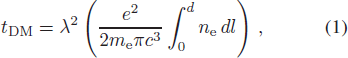where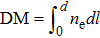is the ‘dispersion measure (DM)’, λ is the observing wavelength, c is the speed of light, e is the electron charge, m e is the mass of an electron and d is the distance between the Earth and the pulsar. The DM at a given time can be determined if pulse arrival times have been measured at multiple observing frequencies. The precision with which the DM can be measured is determined by the arrival time uncertainties and the frequency coverage of the observations. If the DM variations can be measured with sufficient precision then such variations can be accounted for in the pulsar timing model. Methods to do this for the PPTA sample of pulsars were described by You et al. (), Keith et al. () and Reardon et al. () 2 . These papers showed that the DM variations can often, but not always, be modelled by assuming Kolmogorov turbulence in the interstellar medium. However, Keith et al. () showed that, without care, the method used to account for DM variations can remove an underlying gravitational wave signal. By simultaneously modelling the frequency-dependent signal (the DM variations) along with a frequency-independent signal (known as the “common mode” and would include the gravitational wave signal), it was shown that the gravitational wave signal would not be affected. This method works well when DM variations are relatively smooth and changes occur over the timescale of many observations (i.e., weeks to months).

You et al. () and You et al. () studied observations of PPTA pulsars whose line-of-sight passes close to the Sun. They demonstrated that, at an observing frequency of 1400 MHz, the solar wind can contribute ToA errors of 100 ns for sources 60° from the Sun and more than 1 μs within 7°. In contrast to variations caused by turbulence in the interstellar medium, DM variations caused by the solar wind depend strongly on the line-of- sight angle to the Sun and change on various timescales including day-to-day variability and multi-year variability because of the solar cycle.

Standard pulsar timing software packages such as tempo and tempo2 (Hobbs et al. ) include simple models to account for the solar wind. The default model is a time-independent, spherically symmetric model completely defined by the angle of the line-of-sight to the Sun and a single electron density parameter (NE1AU: the electron density at 1AU). You et al. () demonstrated that this parameterisation was not suitable for modelling the actual observations 3 . You et al. () attempted to model the measured DM changes using observations from the Wilcox Solar Observatory 4 (WSO). WSO models do predict variations in DM changes caused by the solar wind that are similar to what we observe, but as shown by You et al. (), they are not sufficient for correcting a given pulsar data set.

To date, PPTA observations have been carried out either with a relatively small bandwidth centred in the 20 cm observing band, or using a dual-band (10/50 cm) receiver. In a typical observing session, a given pulsar is first observed using one of these receivers and then (perhaps a few days later) observed using the other receiver. Although this is not regularly done, we can also obtain estimates of a pulsar’s DM at a given time using low frequency telescopes, such as the Murchison Widefield Array (MWA) (Bhat et al. ) 5 . This provides very low frequency (80-300 MHz) observations, but not necessarily at the same time as the Parkes observations. We are also currently commissioning an ultra-wide-bandwidth receiver for Parkes that should enable us to simultaneously observe from 700 MHz to 3 GHz. Many other observatories are also building ultra-wide-bandwidth receivers. For instance, the Five-hundred-meter Aperture Spherical Telescope (FAST) 6 will have a receiver providing a band from 270 MHz to 1620 MHz (Zhang et al. ).

To date, PPTA observations have been carried out either with a relatively small bandwidth centred in the 20 cm observing band, or using a dual-band (10/50 cm) receiver. In a typical observing session, a given pulsar is first observed using one of these receivers and then (perhaps a few days later) observed using the other receiver. Although this is not regularly done, we can also obtain estimates of a pulsar’s DM at a given time using low frequency telescopes, such as the Murchison Widefield Array (MWA) (Bhat et al. ) 5 . This provides very low frequency (80-300 MHz) observations, but not necessarily at the same time as the Parkes observations. We are also currently commissioning an ultra-wide-bandwidth receiver for Parkes that should enable us to simultaneously observe from 700 MHz to 3 GHz. Many other observatories are also building ultra-wide-bandwidth receivers. For instance, the Five-hundred-meter Aperture Spherical Telescope (FAST) 6 will have a receiver providing a band from 270 MHz to 1620 MHz (Zhang et al. ).

In this paper, we:

Demonstrate how realistic solar wind effects can be simulated within the tempo2 software package;

simulate (1) realistic observations for the ultra-wide-band receiver with the FAST telescope and (2) observations in the low-frequency band with MWA and in the 20 cm band with the Parkes telescope;

trial different methods for measuring and removing the DM variations and test whether any underlying gravitational wave signal would be affected;

discuss the implications of these results for FAST and the PPTA.

## 2 Simulation

We used the ptaSimulate software package to simulate pulsar parameters and ToAs. This software package was developed in order to make realistic simulations of PTA data sets. For our work, we choose to make a simple simulation of the radiometer noise (leading to errors in the ToAs), but include realistic simulations of isotropic, stochastic gravitational waves and of the solar wind. For the radiometer noise we simply assume that the ToA uncertainties are 100 ns which is typical of a “good” pulsar in the PPTA. For the gravitational waves we use the routines described by Hobbs et al. () to simulate a background defined by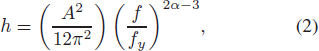where α = −2/3, fy = 1/yr and we choose A = 10−14. This amplitude is much larger than our current upper bounds (Shannon et al. ), but is used to demonstrate the possibility that the gravitational wave signal can be affected by the correction method used to model the solar wind. The solar wind is modelled based on the tempo2 implementation described in You et al. () which uses WSO data as input. We have simulated various pulsars in the PPTA sample, but for this work, we concentrate on PSR J1022+1001 which has the lowest ecliptic latitude (−0.06°) of the pulsars in the sample. We use pta Simulate to provide three noise models: (N1) includes the radiometer noise and gravitational waves, (N2) the radiometer noise and the solar wind and (N3) all three noise processes.

For each of the noise processes we make two data sets:

(DS1): we simulate the FAST telescope and the ultra-wide-band receiver covering a range from 270 to 1620 MHz and we assume that we obtain a single ToA for every 300 MHz interval.

(DS2): we simulate the Parkes telescope observing in 20 cm band and the MWA telescope observing in the lower band.

As shown in Figure 1, for DS1, we simulate 270, 570, 870, 1170 and 1470 MHz as suggested by table 2 in Zhang et al. (). We assume that we obtain a single ToA for every 300MHz interval 7 . For DS2, we simulate 150 and 180 MHz for MWA according to table 1 in Tingay et al. () and table 1 in Loi et al. () and 1300, 1400 and 1500 MHz for Parkes according to table 1 in Manchester et al. ().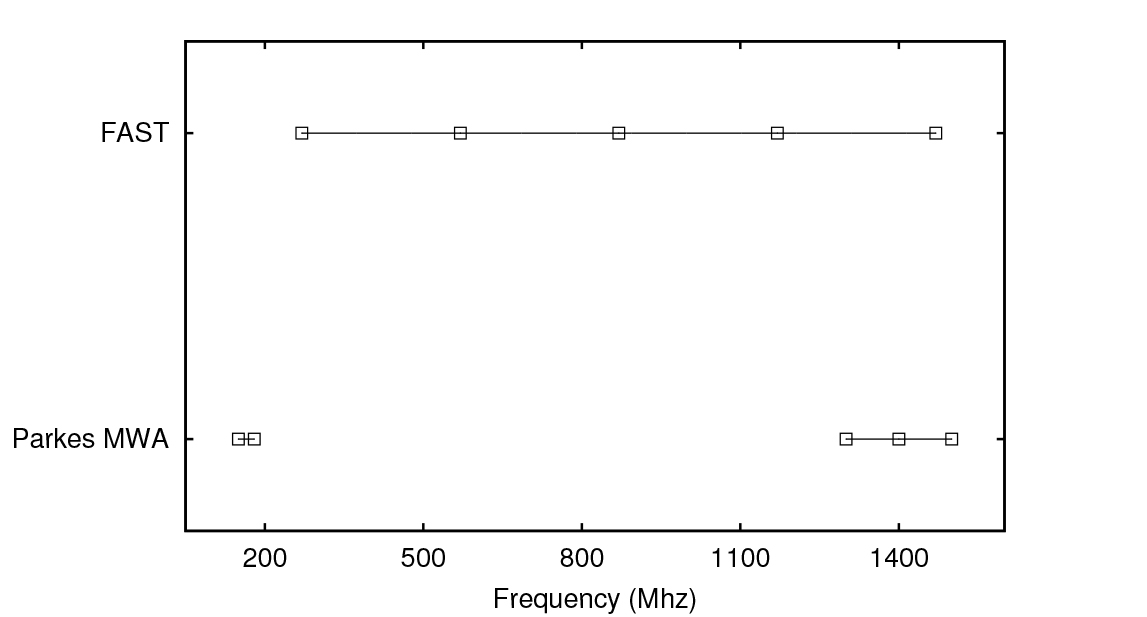Fig. 1 The simulated frequency range for each telescope. Black bars indicate the simulated frequency range and squares indicate the exact frequencies used in the simulation.
Pulsar Ecliptic latitude Peak amplitude for 270 MHz Peak amplitude for 1470 MHz
(°) (μs) (μs)
(1) (2) (3) (4)
J1713+0747 30.70 6.75 0.29
J1744−1134 11.81 22.5 0.9
J1911−1114 11.09 31.8 1.2
J1939+2134 42.30 7.7 0.32
J2229+2643 33.29 10.4 0.51
Notes: The second column is their ecliptic latitude. The third and fourth columns are the peak amplitude of the solar wind signal in our simulation for the low frequency (270 MHz) data and high frequency (1470 MHz), respectively.

Table 1 A List of Pulsars that FAST is Expected to Time with 100 ns Timing Precision

Even though the Parkes and MWA observations provide a frequency span from 80 to 3100 MHz, it is not common for both telescopes to observe simultaneously. Therefore, for DS2 we have also tried different time offsets between the MWA and Parkes observations: (TO1) the observations were simultaneous; (TO2) the MWA observation occurred one day after the Parkes observations. All of our simulated data sets have a data span of ten years and an observing cadence of 14 d. We simulated from 2005 to 2015 as we use real WSO data sets for the solar wind modelling - clearly the actual FAST and Parkes/MWA data do not cover this time range, but the results will not depend upon the exact dates chosen.

In order to illustrate the effect of the solar wind, we have also simulated the highest and lowest (270 MHz and 1470 MHz respectively) observing bands for FAST, but with a 1 d sampling.

In Figure 2(a) we show a typical realisation of the gravitational wave background signal over the 10 year data span. in Figure 2(b) we show the simulated signal with the gravitational wave background and the solar wind. The solar wind clearly produces timing residuals many orders of magnitude larger than the gravitational wave signal. As expected, the signal has an annual periodicity representing the times when the line- of-sight to the pulsar goes close to the Sun. The exact signal depends upon the solar cycle and the exact data sampling. In insets (b1) and (b2) of Figure 2(b) we show an enlarged view around year 2008 for 1470 MHz and 270 MHz. In this panel we have not attempted to remove the effect of the solar wind using any method. In Figure 2(c) we show the same data, but, this time, use the standard tempo/tempo2 spherically symmetric solar wind model in which we assume that the electron density at 1 AU is 10 e cm−3. Clearly, significant (both positive and negative) residuals are still seen and so this simple model both over-corrects and under-corrects the data at different epochs and does not provide a sufficient means to remove the solar wind variations.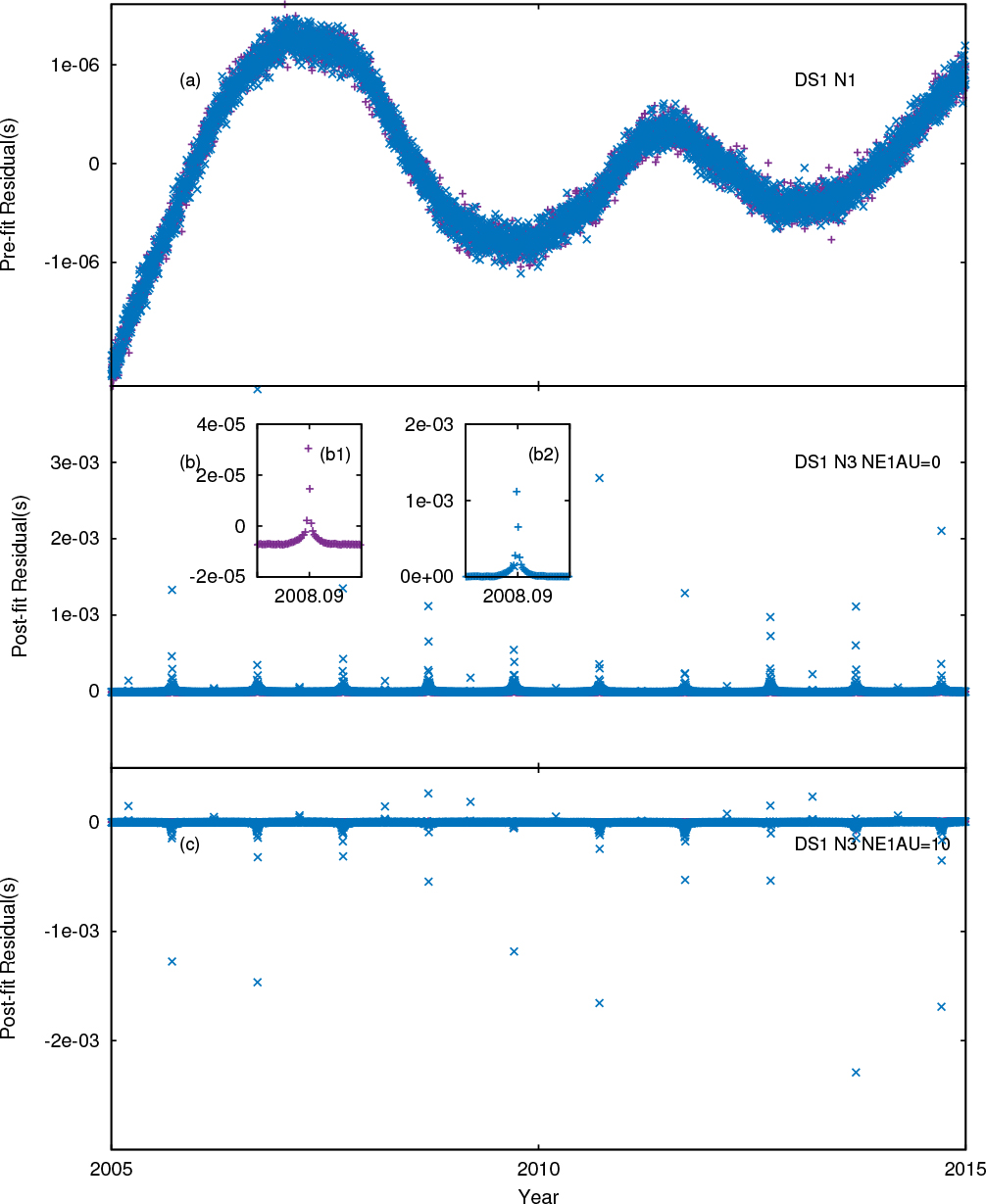Fig. 2 Simulated residuals for FAST. Purple crosses mark simulated frequency 1470 MHz and blue crosses mark simulated frequency 270 MHz. The top panel (a) shows expected white radiometer noise and the low frequency gravitational wave signal. The central panel (b) shows radiometer noise and the effect of the solar wind. Panel (c) shows the same, but after attempting to model the solar wind using a simple, spherically-symmetric model.

## 3 Method and Results

In Figure 3, we show the power spectral density of the pre-fit residuals for DS2. The green line shows the spectral density for the timing residuals affected by the solar wind and radiometer noise. The purple line is the same, but for the simulation containing only gravitational wave and radiometer noise. The dotted line is the analytic representation of the power spectrum of the simulated gravitational waves. Producing a single spectrum using an identical method is challenging in this case as the gravitational wave signal has a steep power-law signal which requires a pre-whitening method to mitigate the effects of spectral leakage. However, the solar wind signal does not follow a steep power-law spectrum and therefore we should not use pre-whitening methods. The goal of our analysis here is to remove the effect of the solar wind without removing the gravitational wave signal. We therefore present these power spectra in a way that is optimal for the steep gravitational wave power law spectrum and note that the spectra of the solar wind will not be perfect (particularly at low frequencies). Throughout this paper we therefore input a set of pulsar timing residuals and form a power spectrum assuming a gravitational wave background power law spectrum using the “analyticChol” and “cholSpectra” tempo2 plugins 8 . Note that this same procedure was followed by Tiburzi et al. (). Clearly, without correction the solar wind effect dominates the entire spectrum and makes the gravitational waves undetectable. Our aim in this section is to determine whether existing methods can be used to remove the effect of the solar wind, without also removing the gravitational wave signal.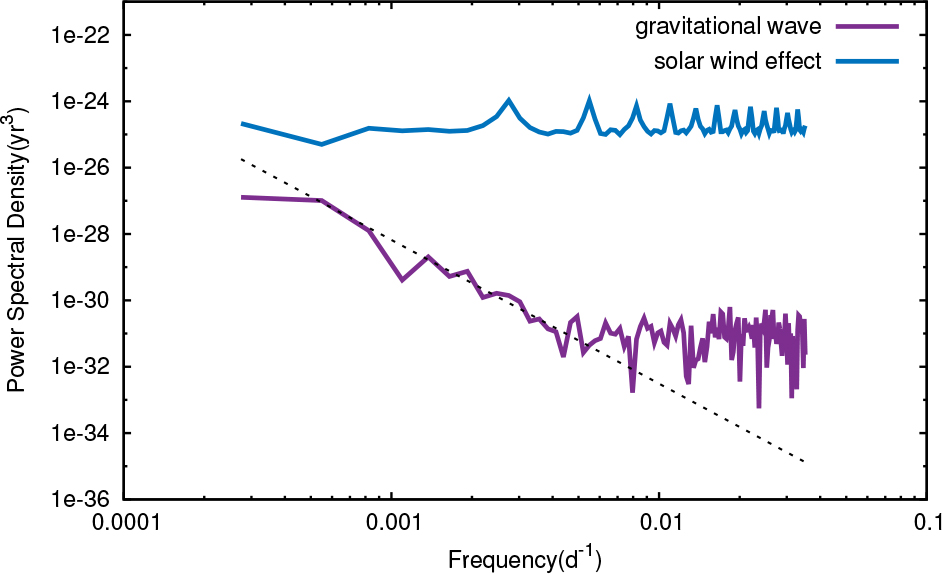Fig. 3 Power spectral density of pre-fit residuals. The blue line indicates the simulated solar wind effect and radiometer noise. The purple line marks the simulated gravitational wave and radiometer noise. The dotted line is the simulated power law model of the gravitational wave background signal.

In order to mitigate the effect of DM variations caused by the interstellar medium, Keith et al. () used the simultaneous fitting of a frequency-dependent and frequency-independent function to the residuals. The function is sampled at specified time intervals. The user is able to choose the time sampling, but they reported optimal time intervals to correct DM variations caused by the interstellar medium. These optimal time intervals are long and, for the PPTA pulsars, are listed in their table 2. We have trialled 30, 100, 200 and 300 d samplings. However, as seen from the inset of Figure 2(b), the solar wind induces timing residuals on much shorter timescales. As we expected (and shown in Fig. 4), the correction is poor 9 . It is clear that the solar wind features in the power spectrum have not been removed and the power spectrum remains orders-of-magnitude higher than the power spectrum of the simulated gravitational wave signal.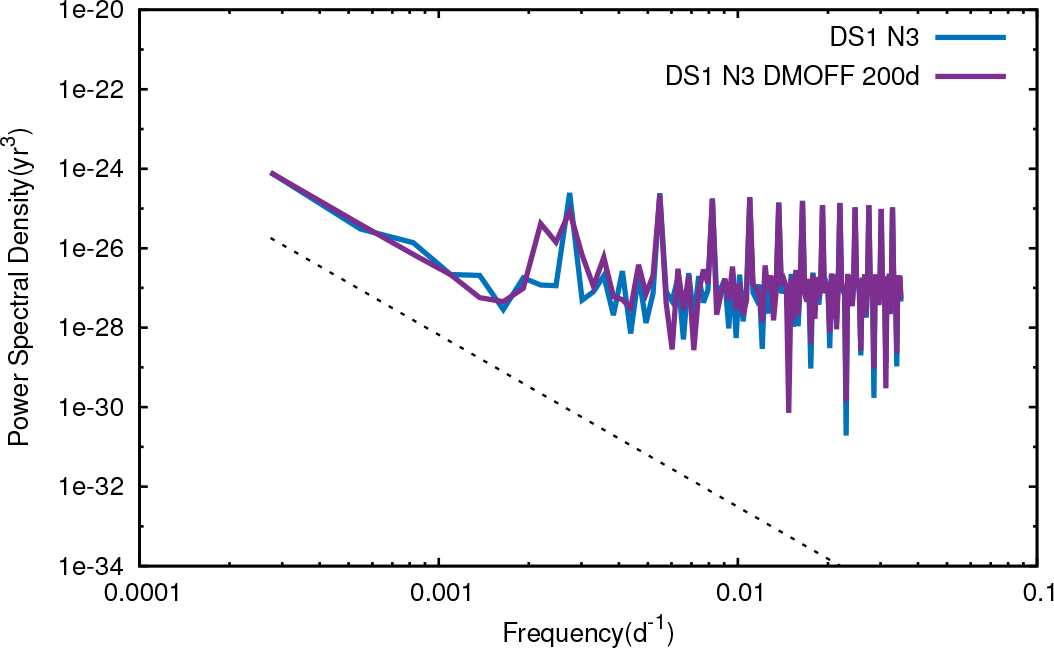Fig. 4 Power spectral density for the simulated N3 data set for FAST. The purple line is the power spectral density of pre-fit residuals and the blue line is the post-fit residuals after fitting a frequency-dependent and common-mode signal using a 300 d sample. The dotted line is the simulated power law model of the gravitational wave background signal.

The Keith et al. () method does not require a common gridding between the frequency-dependent and frequency-independent functions. We expect that the frequency-dependent function (representing the solar wind) will vary quickly, but the frequency-independent function (such as the gravitational wave signal) can be sampled on a much sparser grid. We therefore fitted using a grid spacing of 10 d for the DM (frequency-dependent) function combined with a 300 d sampling for the common mode (frequency-independent) function.

We trialled this method on both DS1 and DS2 and present the resulting power spectra in Figure 5. We demonstrate that using a sampling (14 d) for the frequency-dependent function implies that we can model any DM variations occurring during a single observing epoch. We simultaneously fit a 300 d frequency-independent function.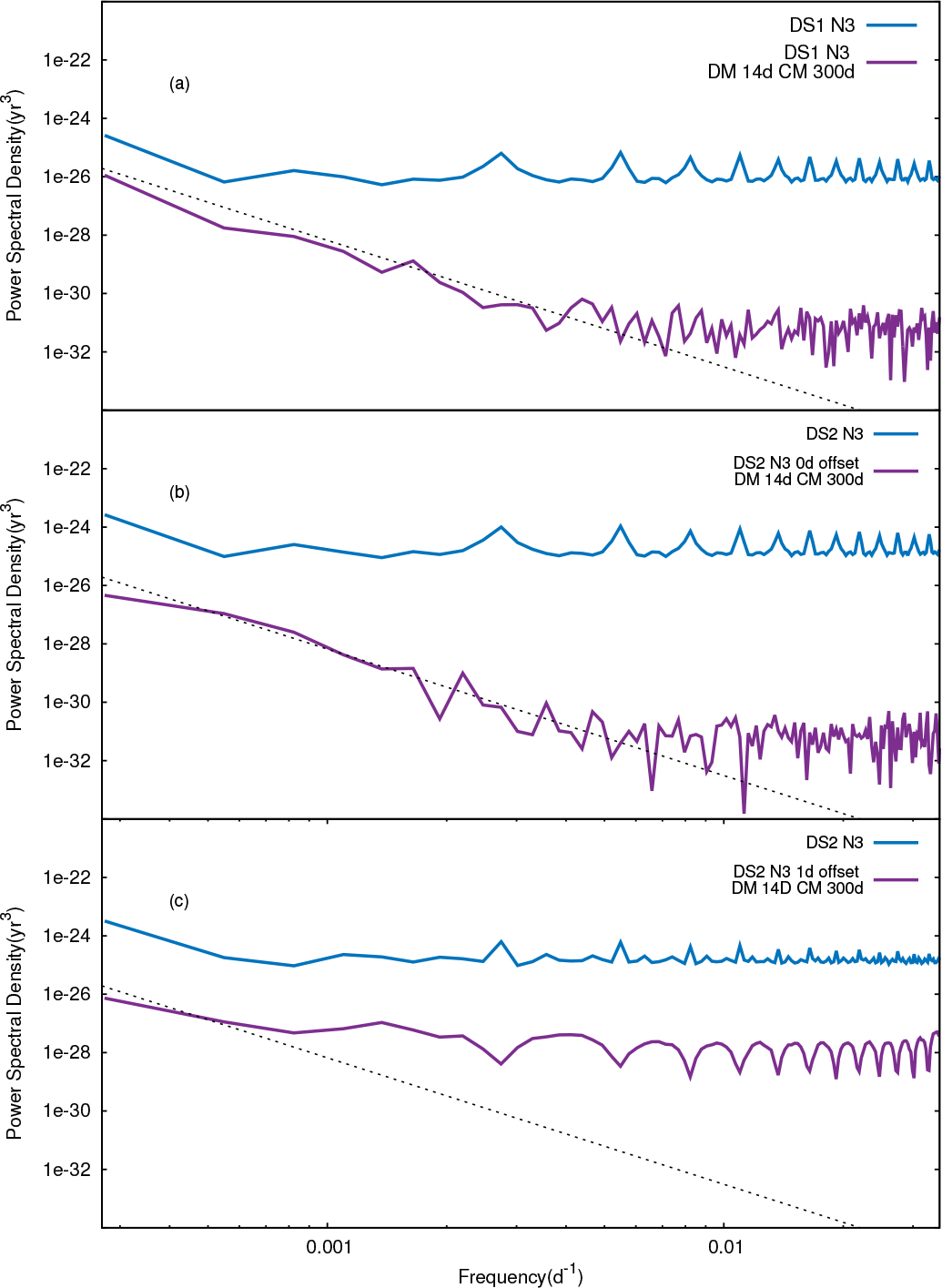Fig. 5 Pre-fit (purple) and post-fit (blue) power spectral densities for (a) a simulation of FAST, (b) Parkes/MWA without any time offset and (c) Parkes/MWA with a 1 d time offset. The dotted line is the simulated power law model of the gravitational wave background signal.

In Figure 5(a) we present the pre- and post-fit spectra for our simulation of FAST (DS1) 10 . In Figure 5(b) we show the same, but for the Parkes/MWA data set in which both telescopes are assumed to observe at the same time. Both panels (a) and (b) indicate that this process works well; we can remove the signature of the solar wind without removing the gravitational wave signal. However, in Figure 5(c) we show the resulting power spectra if the Parkes and MWA observations are offset in time by 1 d. In this case it is clear that we are unable to completely remove the solar wind variations (because the solar wind varies on a timescale faster than a day; see figure 5 in You et al. () for a demonstration of this with actual observations). Although we note that the lowest frequency channels in the power spectrum are now at the expected level of the gravitational wave signal, we also highlight that our simulated gravitational wave signal is at least one-order-of-magnitude higher than the currently expected level.

## 4 Discussion and conclusions

Tiburzi et al. () highlighted that when the line-of-sight to pulsars at low ecliptic latitudes passes close to the Sun then the effects of the solar wind can become large. Typically, PTA experiments simply exclude observations within a certain angle from the Sun, but with sufficiently high precision (as will be achievable with telescopes like FAST and the SKA) simply excluding observations will not always be practical. Tiburzi et al. () attempted to model the solar wind using a 100 d gridding, but noted that this was ineffective as the variations caused by solar wind are narrow, cuspy and change from year to year. Other correction/modelling methods, such as Bayesian analysis methods (Lentati et al. ) also assume simplistic models for the solar wind.

Our results indicate that the Keith et al. () procedure can be used to model and remove the solar wind effect assuming that the frequency-dependent function has the same gridding as the observing cadence and a wide-bandwidth receiver is used. This is promising for FAST assuming that the wide-bandwidth receiver is available for high-precision pulsar timing. As the primary goal for early science operations for FAST is to carry out a survey with a multibeam, 20 cm receiver, it is possible that the wide-bandwidth receiver will not be commonly available. In this case it will become much harder to correct for the effect of the solar wind and as shown in the Parkes-MWA simulations it will not be trivial to model the solar wind sufficiently using other telescope observations (unless they are obtained simultaneously with the FAST data).

The sampling required for the solar wind correction is much faster than the optimal sampling given by Keith et al. () for modelling and removing DM variations caused by the interstellar medium. When processing actual PTA data sets it will therefore be necessary to measure the DM variations on an uneven grid spacing. At times when the line of sight to the pulsar goes close to the Sun then a fast sampling will be required. At other times the Keith et al. () sampling will need to be used. As the solar wind is variable and also the interstellar medium variations can have abrupt changes (for instance, Coles et al. () report observations of two extreme scattering events) it is likely that the grid spacing will need to be chosen carefully and manually.

We note that the pulsar we chose in this paper (PSR J1022+1001) is the PTA pulsar that has the smallest ecliptic latitude and therefore the solar wind will have the largest effect for this pulsar. Zhang et al. (in preparation) suggest that five International Pulsar Timing Array (IPTA) pulsars (PSRs J1713+0747, J1744–1134, J1911–1114, J1939+2134 and J2229+2643) are likely to have ToA uncertainties smaller than 100 ns during a typical FAST timing observation. We have re-simulated our daily-sampled FAST data set for these pulsars. Clearly the signal strength amplitude is related to the ecliptic latitude. However, as shown in Table 1, for all pulsars the solar wind effect is detectable in the simulated data sets and for some pulsars (such as PSRs J1911–1114 and J1744–1134) the signal is significantly higher than the 100 ns level.

We also note that the amplitude of gravitational waves we set is much larger than our current upper bounds (Shannon et al. ). To make sure our method is effective for detecting a gravitational wave, we re-simulate data for Figure 5(b) with a lower value of the background amplitude, A = 10−15. The results are shown in Figure 6 and we note that the algorithm presented here works well even with the lower gravitational wave background amplitude.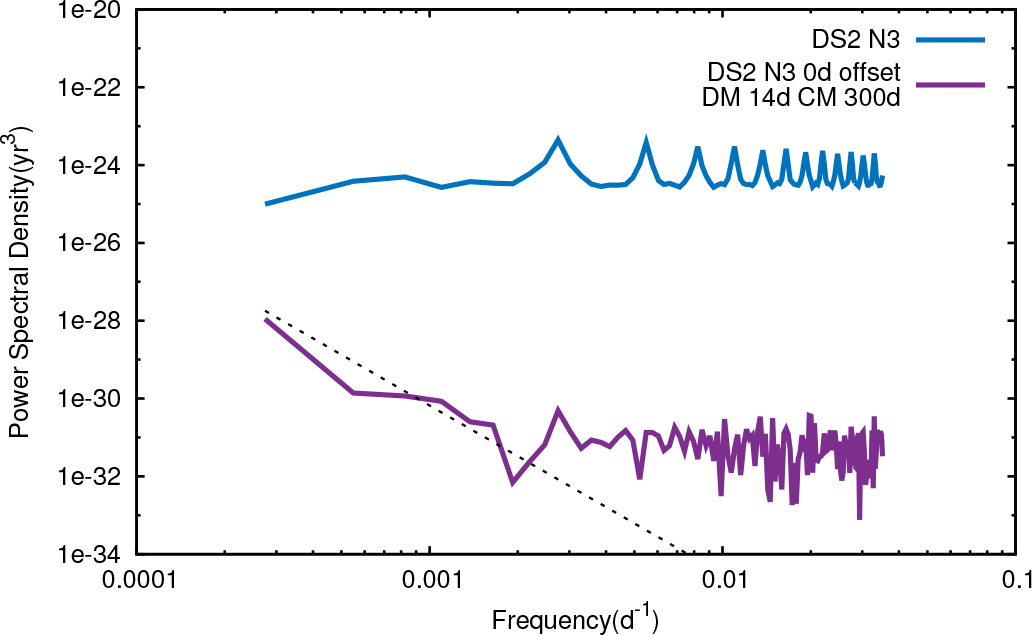Fig. 6 Same figure as Fig. 5(b) but changing the amplitude of gravitational wave background to A = 10−15.

For this paper we have studied the use of the Keith et al. () method for modelling and removing the effect of the solar wind from PTA data sets. Other methods are available. For instance, the “DMX” procedure (e.g., Demorest et al. ) can be used in a similar manner, but the common-mode signal must be accounted for in the fitting process to ensure that the gravitational wave signal is not removed. Similarly, Bayesian methods exist (e.g., Lentati et al. ), but producing a model for the solar wind is non-trivial and, to our knowledge, has not been attempted within the Bayesian frameworks.

In conclusion, the solar wind will induce timing residuals that will need to be modelled and/or removed when analysing PTA data. Our work suggests that this can be done with a wide-enough, simultaneous observing frequency coverage. We also note that the solar wind is intrinsically interesting. Instead of simply removing and discarding the solar wind signature, it can be used (as shown by You et al. ) to improve the accuracy of magnetic field estimates of the solar wind near the Sun.

 Arzoumanian Z. Brazier A. Burke-Spolaor S. et al. 2016 ApJ 821 13 Bhat N. D. R. Ord S. M. Tremblay S. E. et al. 2014 ApJ 791 L32 Coles W. A. Kerr M. Shannon R. M. et al. 2015 ApJ 808 113 Coles W. Hobbs G. Champion D. J. Manchester R. N. Verbiest J. P. W. 2011 MNRAS 418 561 Demorest P. B. Ferdman R. D. Gonzalez M. E. et al. 2013 ApJ 762 94 Desvignes G. Caballero R. N. Lentati L. et al. 2016 MNRAS 458 3341 Dewdney P. E. Hall P. J. Schilizzi R. T. Lazio T. J. L.W. 2009 IEEE Proceedings 97 1482 Hobbs G. B. Edwards R. T. Manchester R. N. 2006 MNRAS 369 655 Hobbs G. Lyne A. G. Kramer M. Martin C. E. Jordan C. 2004 MNRAS 353 1311 Hobbs G. Jenet F. Lee K. J. et al. 2009 MNRAS 394 1945 Keith M. J. Coles W. Shannon R. M. et al. 2013 MNRAS 429 2161 Lam M. T. Cordes J.M. Chatterjee S. et al. 2016 ApJ 821 66 Lentati L. Alexander P. Hobson M. P. et al. 2014 MNRAS 437 3004 Loi S. T. Trott C. M. Murphy T. et al. 2015 Radio Science 50 574 Manchester R. N. Hobbs G. Bailes M. et al. 2013 PASA 30 e017 Reardon D. J. Hobbs G. Coles W. et al. 2016 MNRAS 455 1751 Shannon R. M. Ravi V. Lentati L. T. et al. 2015 Science 349 1522 Tiburzi C. Hobbs G. Kerr M. et al. 2016 MNRAS 455 4339 Tingay S. J. Goeke R. Bowman J. D. et al. 2013 PASA 30 e007 You X. P. Coles W. A. Hobbs G. B. Manchester R. N. 2012 MNRAS 422 1160 You X. P. Hobbs G. B. Coles W. A. Manchester R. N. Han J. L. 2007a ApJ 671 907 You X. P. Hobbs G. Coles W. A. et al. 2007b MNRAS 378 493 Zhang L. Hobbs G. Li D. et al. 2016 RAA (Research in Astronomy and Astrophysics) 16 151
Cite this article: Niu Ze-Xi, Hobbs George, Wang Jing-Bo, Dai Shi. Correcting for the solar wind in pulsar timing observations: the role of simultaneous and low-frequency observations. Cancer Biol Med. 2017; 10:103.

### Refbacks

• There are currently no refbacks.